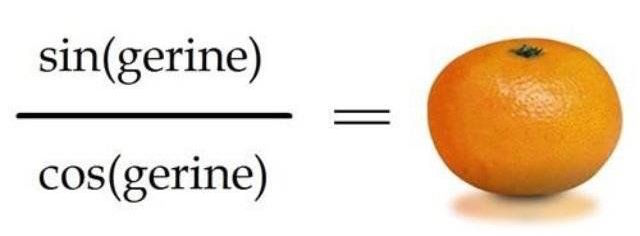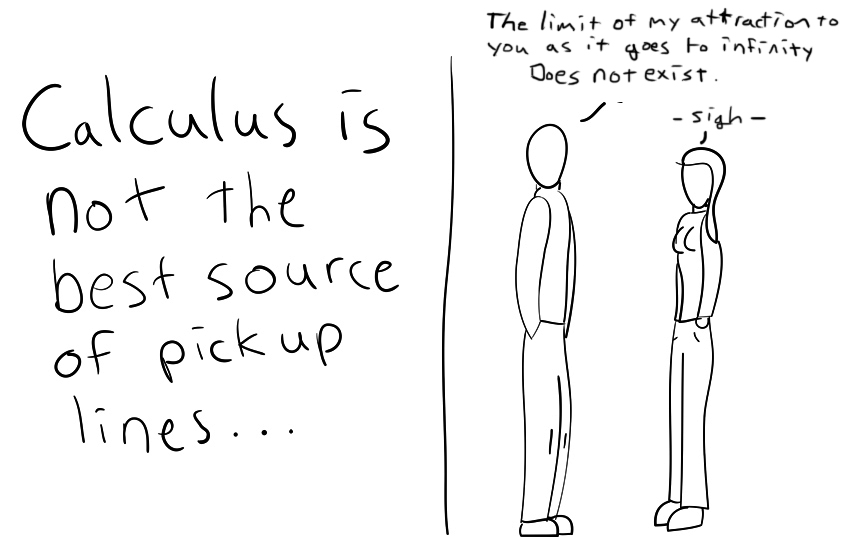# 10 (Extra Cheesy) Math Jokes Explained

If you’re a math teacher looking for some cheesy yet relevant material, a giant math nerd, or simply a lover of lame jokes – check out these punny math images:1. One of the basic trigonometric rules is that Sin(x)/Cos(x) = Tan(x). So, sin(gerine)/cos(gerine) = tan(gerine). Mathematical and refreshing!2. A great joke for your calculus students using old school Pokémon imagery. The derivative of e^x is always e^x.  Plus, any opportunity use Pokémon in the classroom should be taken.3. The number i is an imaginary number, -1 . It is not considered a real number.  A rational number is a number that can be expressed as a fraction.  The decimals of pi go on forever, it is impossible for pi to be represented as a fraction, thus it is not rational.4. To find the volume of a disk, you multiply the area of the base times the depth. The volume is pi * r * r * depth. If r = z, and depth = a, our result is pi * z * z * a.5. A hidden joke from Futurama: Historic √66 (sound it out…).6. What this suave guy was trying to say: my attraction to you has no limit. In calculus limits are the value that a function or sequence “approaches” as the input or index approaches some value.Better luck next time, maybe try a different math pick up line. Though, ladies, this line would work on me.7. In trigonometry, sin^2(x) + cos^2(x) will always equal 1, no matter what the x is. It is based off of the pythagorean theorem. That doesn’t mean this pick up line is any better than that other one…. but hey, maybe its worth a shot. You never know.8. Two math jokes in one! An acute angle is an angle that is less than 90 degrees. Complementary angles are angles two angles that add up to 90 degrees.9. I have to include something xkcd.  The beautiful thing about math is that there are universal rules that apply to all situations.  There is no room for debate.

“a(b+c) = ab + ac, politicize that, b******.”

– Randall Munroe (author of the comic)10. A normal distribution is a bell curve.  This is what educators use for calculating standard deviations and all sorts of other fun things!!!  The paranormal distribution on the other hand is 1+1 = 2 spooky 4 me.

Have a funny favorite we missed? Let us know in the comments!

Also, visit the math Homework Help section on eNotes and get help with your own homework problems.